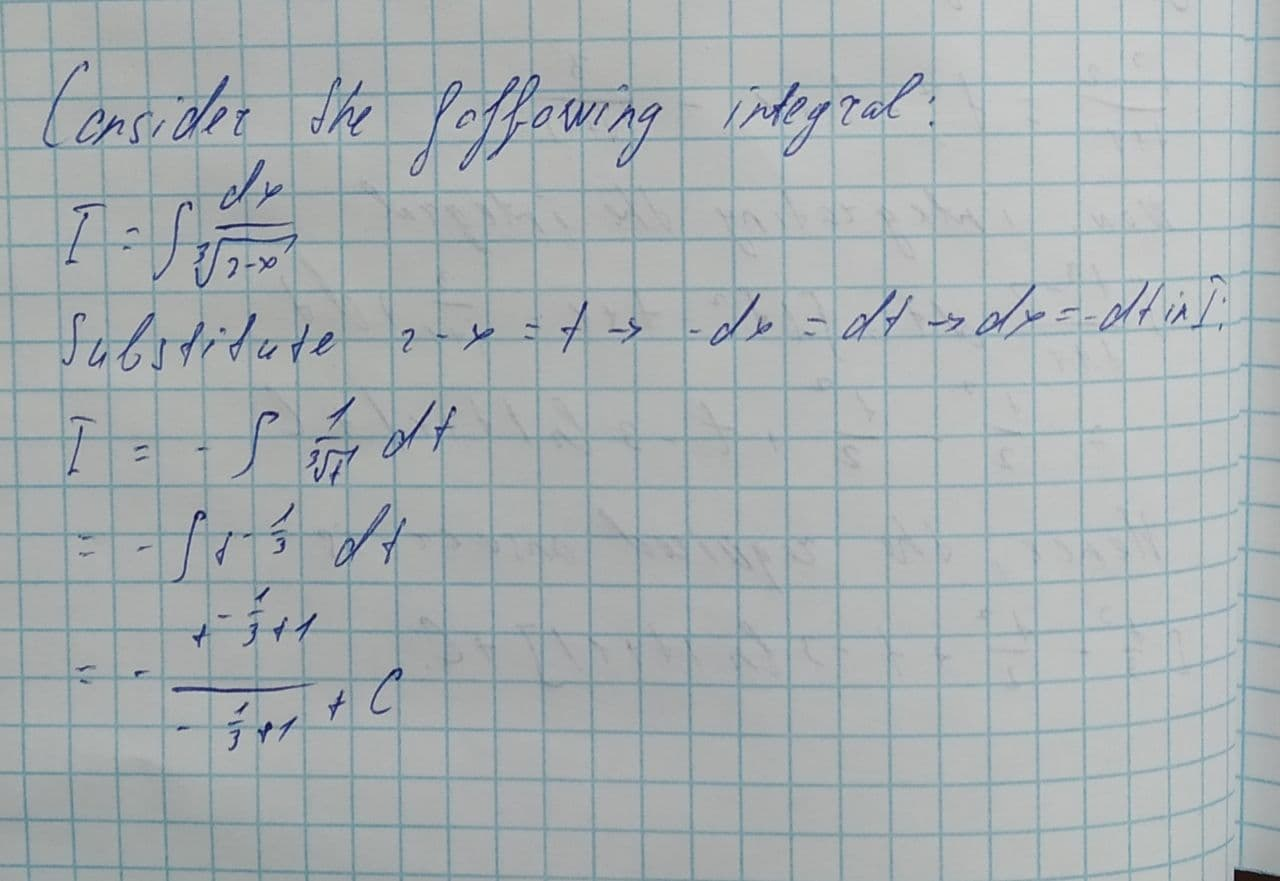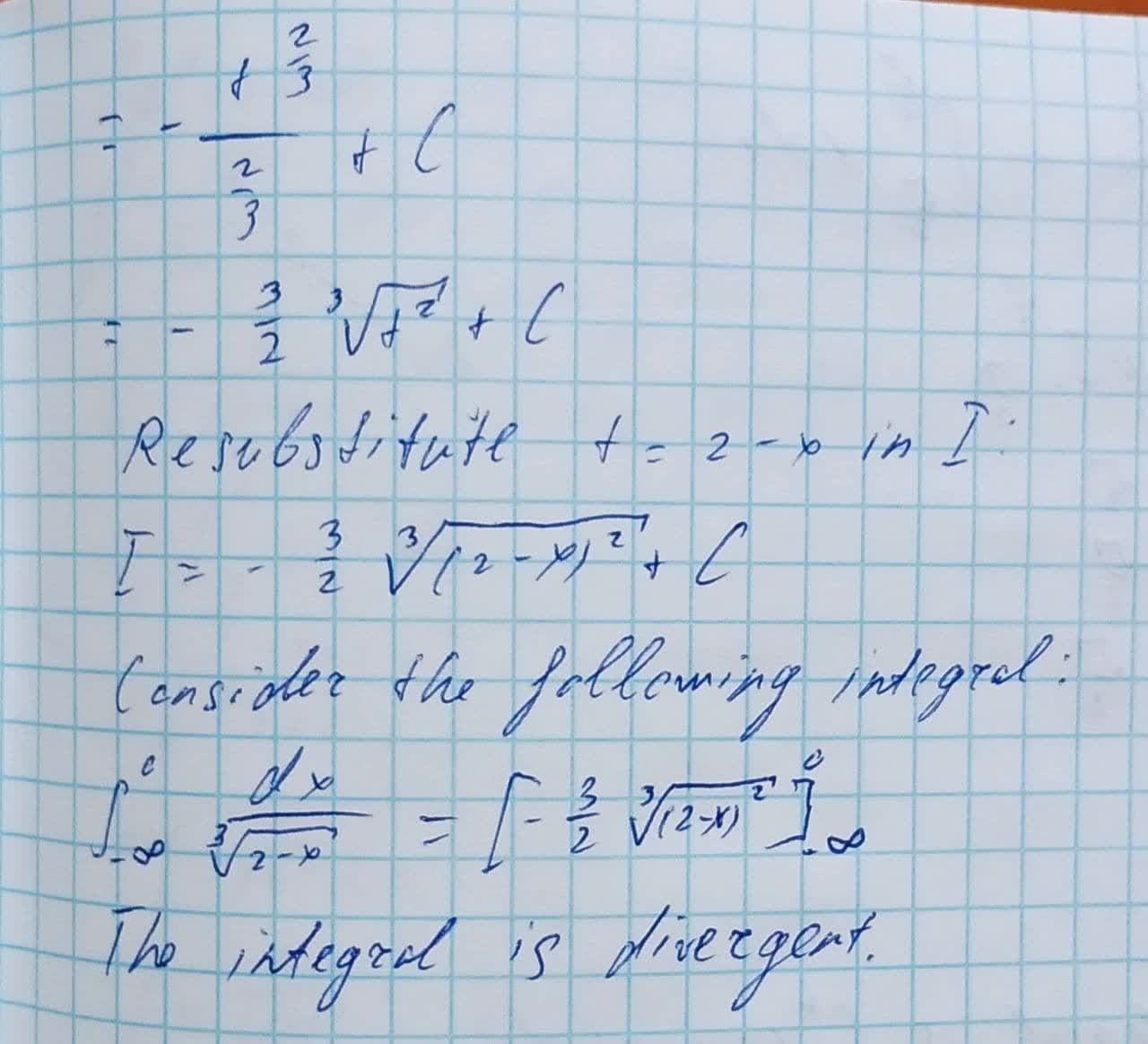Question# Evaluate the following integrals or state that they diverge \int_{-\infty}^{0}\frac{dx}{\sqrt{2-x}}

Applications of integrals
ANSWEREDEvaluate the following integrals or state that they diverge.
$$\displaystyle{\int_{{-\infty}}^{{{0}}}}{\frac{{{\left.{d}{x}\right.}}}{{\sqrt{{{3}}}{\left\lbrace{2}-{x}\right\rbrace}}}}$$